2015/02/11 13:52

### cube

Creates a cube at the origin of the coordinate system. When center is true the cube will be centered on the origin, otherwise it is created in the first octant. The argument names are optional if the arguments are given in the same order as specified in the parameters

Parameters

• size

• Decimal or 3 value array. If a single number is given, the result will be a cube with sides of that length. If a 3 value array is given, then the values will correspond to the lengths of the X, Y, and Z sides. Default value is 1.

• center

• Boolean. This determines the positioning of the object. If true, object is centered at (0,0,0). Otherwise, the cube is placed in the positive quadrant with one corner at (0,0,0). Defaults to false

Usage examples:

cube(size = 1, center = false);
cube(size = [1,2,3], center = true);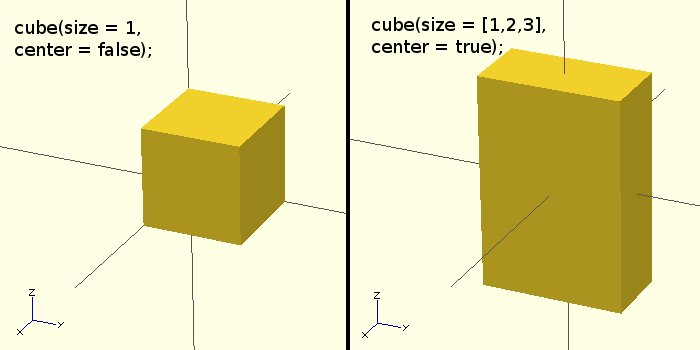### sphere

Creates a sphere at the origin of the coordinate system. The argument name is optional.

Parameters

• Radius. This is the radius of the sphere. The resolution of the sphere will be based on the size of the sphere and the $fa,$fs and $fn variables. For more information on these special variables look at: OpenSCAD_User_Manual/Other_Language_Features • Diameter. This is the diameter of the sphere. (NOTE: d is only available in versions later than 2014.03. Debian is currently known to be behind this) •$fa

• Fragment angle in degrees

• $fs • Fragment size in mm •$fn

• Resolution

Usage Examples

sphere(r = 1);
sphere(r = 5);
sphere(r = 10);
sphere(d = 2);
sphere(d = 10);
sphere(d = 20);

// this will create a high resolution sphere with a 2mm radius
sphere(2, $fn=100); // will also create a 2mm high resolution sphere but this one // does not have as many small triangles on the poles of the sphere sphere(2,$fa=5, $fs=0.1);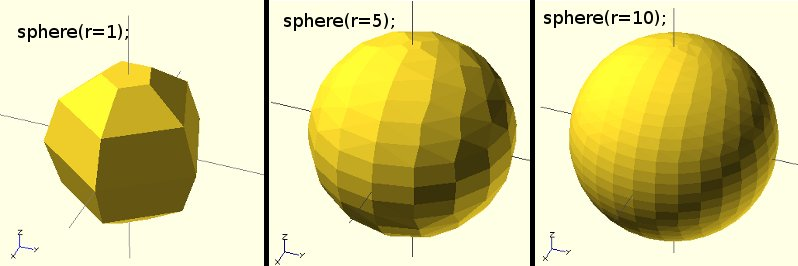### cylinder Creates a cylinder or cone at the origin of the coordinate system. A single radius (r) makes a cylinder, two different radi (r1, r2) make a cone. Parameters • Decimal. This is the height of the cylinder. Default value is 1. • Decimal. The radius of both top and bottom ends of the cylinder. Use this parameter if you want plain cylinder. Default value is 1. • r1 • Decimal. This is the radius of the cone on bottom end. Default value is 1. • r2 • Decimal. This is the radius of the cone on top end. Default value is 1. • Decimal. The diameter of both top and bottom ends of the cylinder. Use this parameter if you want plain cylinder. Default value is 1. • d1 • Decimal. This is the diameter of the cone on bottom end. Default value is 1. • d2 • Decimal. This is the diameter of the cone on top end. Default value is 1. • center • boolean. If true will center the height of the cone/cylinder around the origin. Default is false, placing the base of the cylinder or r1 radius of cone at the origin. •$fa

• The angle (in degrees) from one fragment to the next. See OpenSCAD_User_Manual/Other_Language_Features.

• $fs • The circumferential length of each fragment. See OpenSCAD_User_Manual/Other_Language_Features. •$fn

• The fixed number of fragments to use. See OpenSCAD_User_Manual/Other_Language_Features.

(NOTE: d,d1,d2 are only available in version later than 2014.03. Debian is currently know to be behind this)

Usage Examples

cylinder(h = 10, r=20);
cylinder(h = 10, r=20, $fs=6); cylinder(h = 10, r1 = 10, r2 = 20, center = false); cylinder(h = 10, r1 = 20, r2 = 10, center = true); cylinder(h = 10, d=40); cylinder(h = 10, d=40,$fs=6);
cylinder(h = 10, d1 = 20, d2 = 40, center = false);
cylinder(h = 10, d1 = 40, d2 = 20, center = true);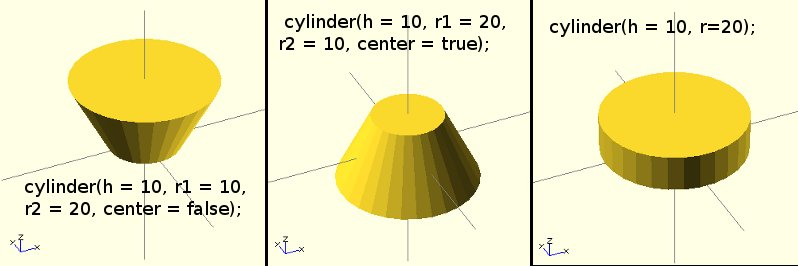### polyhedron

Create a polyhedron with a list of points and a list of faces. The point list is all the vertices of the shape, the faces list is how the points relates to the surfaces of the polyhedron.

note: if your version of OpenSCAD is lower than 2014.03 replace "faces" with "triangles" in the below examples

Parameters

• points

• vector of points or vertices (each a 3 vector).

• triangles

• (deprecated in version 2014.03, use faces) vector of point triplets (each a 3 number vector). Each number is the 0-indexed point number from the point vector.

• faces

• (introduced in version 2014.03) vector of point n-tuples with n >= 3. Each number is the 0-indexed point number from the point vector. That is, faces=[[0,1,4]] specifies a triangle made from the first, second, and fifth point listed in points. When referencing more than 3 points in a single tuple, the points must all be on the same plane.

• convexity

• Integer. The convexity parameter specifies the maximum number of front sides (back sides) a ray intersecting the object might penetrate. This parameter is only needed for correctly displaying the object in OpenCSG preview mode and has no effect on the polyhedron rendering.

Syntax example

 polyhedron(points = [ [x, y, z], ... ], faces = [ [p1, p2, p3..], ... ], convexity = N);

Point ordering for faces When looking at the face from the outside inwards, the points must be clockwise. You can rearrange the order of the points or the order they are referenced in each tuple. The order of faces is immaterial. Note that if your polygons are not all oriented the same way OpenSCAD will either print an error or crash completely, so pay attention to the vertex ordering. Again, remember that the 'pN' components of the faces vector are 0-indexed references to the elements of the points vector.

Example, a square base pyramid:

polyhedron(
points=[ [10,10,0],[10,-10,0],[-10,-10,0],[-10,10,0], // the four points at base
[0,0,10]  ],                                 // the apex point
faces=[ [0,1,4],[1,2,4],[2,3,4],[3,0,4],              // each triangle side
[1,0,3],[2,1,3] ]                         // two triangles for square base
);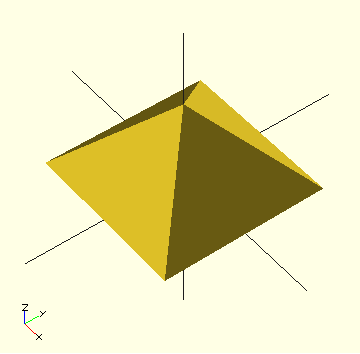A simple polyhedron, square based pyramid

An example of a more complex polyhedron, and showing how to fix polyhedrons with badly oriented polygons.

When you select 'Thrown together' from the view menu and compile the design (not compile and render!) you will see a preview with the mis-oriented polygons highlighted. Unfortunately this highlighting is not possible in the OpenCSG preview mode because it would interfere with the way the OpenCSG preview mode is implemented.)

Below you can see the code and the picture of such a problematic polyhedron, the bad polygons (faces or compositions of faces) are in pink.

// Bad polyhedronpolyhedron    (points = [
[0, -10, 60], [0, 10, 60], [0, 10, 0], [0, -10, 0], [60, -10, 60], [60, 10, 60],
[10, -10, 50], [10, 10, 50], [10, 10, 30], [10, -10, 30], [30, -10, 50], [30, 10, 50]
],
faces = [
[0,2,3],   [0,1,2],  [0,4,5],  [0,5,1],   [5,4,2],  [2,4,3],                  [6,8,9],  [6,7,8],  [6,10,11], [6,11,7], [10,8,11],		  [10,9,8], [0,3,9],  [9,0,6], [10,6, 0],  [0,4,10],                  [3,9,10], [3,10,4], [1,7,11],  [1,11,5], [1,7,8],
[1,8,2],  [2,8,11], [2,11,5]
]
);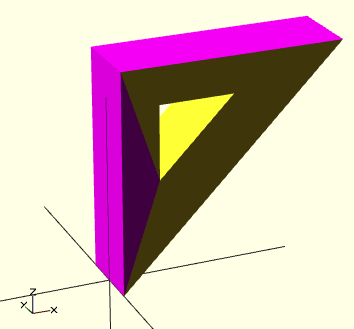A correct polyhedron would be the following:

polyhedron    (points = [
[0, -10, 60], [0, 10, 60], [0, 10, 0], [0, -10, 0], [60, -10, 60], [60, 10, 60],
[10, -10, 50], [10, 10, 50], [10, 10, 30], [10, -10, 30], [30, -10, 50], [30, 10, 50]
],
faces = [
[0,3,2],  [0,2,1],  [4,0,5],  [5,0,1],  [5,2,4],  [4,2,3],                  [6,8,9],  [6,7,8],  [6,10,11],[6,11,7], [10,8,11],		  [10,9,8], [3,0,9],  [9,0,6],  [10,6, 0],[0,4,10],                  [3,9,10], [3,10,4], [1,7,11], [1,11,5], [1,8,7],
[2,8,1],  [8,2,11], [5,11,2]
]
);

Beginner's tip:

If you don't really understand "orientation", try to identify the mis-oriented pink faces and then permute the references to the points vectors until you get it right. E.g. in the above example, the third triangle ([0,4,5]) was wrong and we fixed it as [4,0,5]. In addition, you may select "Show Edges" from the "View Menu", print a screen capture and number both the points and the faces. In our example, the points are annotated in black and the faces in blue. Turn the object around and make a second copy from the back if needed. This way you can keep track.

Clockwise Technique:

Orientation is determined by clockwise indexing. This means that if you're looking at the triangle (in this case [4,0,5]) from the outside you'll see that the path is clockwise around the center of the face. The winding order [4,0,5] is clockwise and therefore good. The winding order [0,4,5] is counter-clockwise and therefore bad. Likewise, any other clockwise order of [4,0,5] works: [5,4,0] & [0,5,4] are good too. If you use the clockwise technique, you'll always have your faces outside (outside of OpenSCAD, other programs do use counter-clockwise as the outside though).

Think of it as a Left Hand Rule:

If you hold the face and the fingers of your hand curls is the same order as the points, then your thumb points outwards.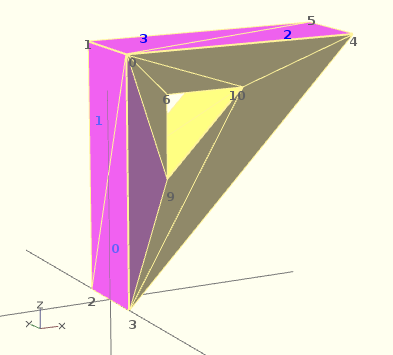Succinct description of a 'Polyhedron'

* Points define all of the points/vertices in the shape.
* Faces is a list of flat polygons that connect up the points/vertices.

Each point, in the point list, is defined with a 3-tuple x,y,z position specification. Points in the point list are automatically given an identifier starting at zero for use in the faces list (0,1,2,3,... etc).

Each face, in the faces list, is defined by selecting 3 or more of the points (using the point identifier) out of the point list.

e.g. faces=[ [0,1,2] ] defines a triangle from the first point (points are zero referenced) to the second point and then to the third point.

When looking at any face from the outside, the face must list all points in a clockwise order.

##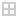GeneralThe text in its current form is incomplete.

## Introduction

OpenSCAD is a 2D/3D and solid modeling program which is based on a Functional programming language used to create models that are later previewed on the screen, and rendered into 3D mesh which allows the model to be exported to a variety of 2D/3D file formats.

Comments are a way of leaving notes within the code (either to yourself or to future programmers) describing how the code works, or what it does. Comments are not evaluated by the compiler, and should not be used to describe self-evident code.

// This is a comment
myvar = 10; // The rest of the line is a comment
/*
can span multiple lines.
*/

### Values and Data Types

A value in OpenSCAD is either a Number (like 42), a Boolean (like true), a String (like "foo"), a Vector (like [1,2,3]), or the Undefined value (undef). Values can be stored in variables, passed as function arguments, and returned as function results.

[OpenSCAD is a dynamically typed language with a fixed set of data types. There are no type names, and no user defined types. Functions are not values. In fact, variables and functions occupy disjoint namespaces.]

#### Numbers

Numbers are the most important type of value in OpenSCAD, and they are written in the familiar decimal notation used in other languages. Eg, -1, 42, 0.5, 2.99792458e+8. [OpenSCAD does not support octal or hexadecimal notation for numbers.]

In additional to decimal numerals, the following names for special numbers are defined:

• PI

OpenSCAD has only a single kind of number, which is an IEEE floating point number. [OpenSCAD does not distinguish integers and floating point numbers as two different types, nor does it support complex numbers.] Because OpenSCAD uses the IEEE floating point standard, there are a few deviations from the behaviour of numbers in mathematics:

• The largest representable number is about 1e308. If a numeric result is too large, then the result can be infinity (printed as inf by echo).

• The smallest representable number is about -1e308. If a numeric result is too small, then the result can be -infinity (printed as -inf by echo).

• If a numeric result is invalid, then the result can be Not A Number (printed as nan by echo).

• If a non-zero numeric result is too close to zero to be representable, then the result will be -0 if the result is negative, otherwise it will be 0. Zero (0) and negative zero (-0) are treated as two distinct numbers by some of the math operations, and are printed differently by 'echo', although they compare equal.

Note that 'inf' and 'nan' are not supported as numeric constants by OpenSCAD, even though you can compute numbers that are printed this way by 'echo'. You can define variables with these values by using:

inf = 1e200 * 1e200;nan = 0 / 0;echo(inf,nan);

Note that 'nan' is the only OpenSCAD value that is not equal to any other value, including itself. Although you can test if a variable 'x' has the undefined value using 'x == undef', you can't use 'x == 0/0' to test if x is Not A Number. Instead, you must use 'x != x' to test if x is nan.

#### Boolean Values

Boolean values are truth values. There are two Boolean values, named 'true' and 'false'. A Boolean value is passed as the 'center' argument to the 'cube' and 'cylinder' primitives, as the argument to 'if', and as the arguments to the logical operators '!' (not), '&&' (and), and '||' (or).

In all of these contexts, you can actually pass any type of value. Most values, when used in a Boolean context, are equivalent to 'true', but there are a few that are equivalent to 'false'. The values that count as false are:

• false

• 0 and -0

• ""

• []

• undef

Note that nan (Not A Number) counts as true.

#### Strings

A string is a sequence of zero or more unicode characters. String values are used to specify file names when importing a file, and to display text for debugging purposes when using 'echo

A string literal is written as a sequence of characters enclosed in quotation marks ("), like this: "", or "this is a string".

To include a " character in a string literal, use \". To include a \ character in a string literal, use \\. The following escape sequences beginning with \ can be used within string literals:

• \" → "

• \\ → \

• \t → tab

• \n → newline

• \r → carriage return

Example:

 echo("The quick brown fox \tjumps \"over\" the lazy dog.\rThe quick brown fox.\nThe \\lazy\\ dog.");

Output:
ECHO: "The quick brown fox jumps "over" the lazy dog.
The quick brown fox.
The \lazy\ dog."

ECHO: "The quick brown fox \tjumps \"over\" the lazy dog.
The quick brown fox.\nThe \\lazy\\ dog."

#### Vectors

A vector is a sequence of zero or more OpenSCAD values. Vectors are most commonly used to represent points in 3-space (as [x,y,z] triples), to represent lists of points, and to represent the size of a cuboid (also as an [x,y,z] triple).

A vector is written as a list of zero or more expressions, separated by commas, and enclosed in square brackets, Eg, [] or [10,20,30].

Example

deck = [64, 89, 18];cube(deck);

Output A cube with the sizes: X = 64, Y = 89, Z = 18.

##### Vectors selection

You can also refer to individual values in a vector with vector[number]. number starts from 0.

Example

deck = [64, 89, 18];translate([0,0,deck]) cube(deck);

Output The same cube as the previous example would be raised by 18 on the Z axis, since vector indices are numbered [0,1,2] for [X,Y,Z] respectively.

##### Matrix

A matrix is a vector of vectors.

Example

mr = [
[cos(angle), -sin(angle)],
[sin(angle),  cos(angle)]
];

Output Define a 2D rotation matrix.

#### Ranges

Ranges define a series of numerical values.

Range: [<start>:<end>] - iterate from start to end inclusive with a fixed increment of 1. Both start and end can be fractions. It also works if end is smaller than start but as of Version 2014.03 this usage is deprecated and will produce a warning message.

Range: [<start>:<increment>:<end>] - iterate from start to end with the given increment. The increment can be a fraction.
It's valid to use a negative increment, in this case end must be smaller than (or equal to) start.

Warning: If the increment is not an even divider of <end>-<start>, the iterator value for the last iteration will be <end>-(<end>-<start> mod <increment>).

Note for Version < 2014.03: The increment is given as an absolute value and cannot be negative. If <end> is smaller than <start> the increment should remain unchanged.

Example

r = [0.5 : 2.5];for (n = r) echo(n);

Output
ECHO: 0.5
ECHO: 1.5
ECHO: 2.5

#### The Undefined Value

The undefined value is a special value written as 'undef'. It's the initial value of a variable that hasn't been assigned a value, and it is often returned as a result by functions or operations that are passed illegal arguments. Finally, 'undef' can be used as a null value, equivalent to 'null' or 'NULL' in other programming languages.

Note that numeric operations may also return nan to indicate an illegal argument. For example, 0/false is undef, but 0/0 is nan. Relational operators like < and > return false if passed illegal arguments.

### Variables

Variables in OpenSCAD are simply an identifier followed by an assignment via an expression (i.e. identifier = expression).

Example:

myvar = 5 + 4;

OpenSCAD is a Functional programming language, as such variables are bound to expressions and keep a single value during their entire lifetime due to the requirements of referential transparency. In imperative languages, such as C, the same behavior is seen as constants, which are typically contrasted with normal variables.

In other words OpenSCAD variables are more like constants, but with an important difference. If variables are assigned a value multiple times, only the last assigned value is used in all places in the code. See further discussion at 'Variables are set at compile-time, not run-time' below. This behavior is due to the need to supply variable input on the command line, via the use of '-D variable=value' option. OpenSCAD currently places that assignment at the end of the source code, and thus must allow a variables value to be changed for this purpose.

The variable retains its last assigned value at compile time, in line with Functional programming languages. Unlike Imperative languages, such a C, OpenSCAD is not an iterative language, as such the concept of x = x + 1 is not valid, get to understand this concept and you will understand the beauty of OpenSCAD.

Currently it's not possible to do assignments at any place (the only places are file top-level and module top-level). So it is invalid inside an if/else or for loop, if you need it inside the for loop you need to use the assign() module.

Update: [Note: Requires version 2014.QX(see )]

In the upcoming version 2014.QX, the restriction on where assignments can be made have been lifted: Variables can now be assigned in any scope. Note that assignments are only valid in the scope in which they are defined - you are still not allowed to leak values to an outer scope.

for (i = [10:50]) {
angle = i*360/20;
distance = i*10;
r = i*2;
rotate(angle, [1, 0, 0])
translate([0, distance, 0])
sphere(r = r);}

Note! Anonymous scopes are not considered scopes:

{
angle = 45;}rotate(angle) square(10);

#### Undefined variable

A non assigned variable has the special value undef. It could be tested in conditional expression, and returned by a function. Example

echo("Variable a is ", a); // output 'Variable a is undef'if (a==undef) {
echo("Variable a is tested undefined");}

Output Variable a is undef Variable a is tested undefined

#### Variables are set at compile-time, not run-time

Because OpenSCAD calculates its variable values at compile-time, not run-time, the last variable assignment will apply everywhere the variable is used (with some exceptions, mentioned below). It may be helpful to think of them as override-able constants rather than as variables.

Example:

 // The value of 'a' reflects only the last set value
a = 0;
echo(a);

a = 5;
echo(a);

Output

ECHO: 5
ECHO: 5

This also means that you can not reassign a variable inside an "if" block:

Example:

a=0;if (a==0)
{
a=1; // <- this line will generate an error.
}

Output Compile Error

##### Exception #1

This behavior is scoped to either the root or to a specific call to a module, meaning you can re-define a variable within a module without affecting its value outside of it. However, all instances within that call will behave as described above with the last-set value being used throughout.

Example:

p = 4;test(5);echo(p);/*
* we start with p = 4.  We step to the next command 'test(5)', which calls the 'test' module.
* The 'test' module calculates two values for 'p', but the program will ONLY display the final value.
* There will be two executions of echo(p) inside 'test' module, but BOTH will display '9' because it is the FINAL
* calculated value inside the module. ECHO: 9   ECHO: 9
*
* Even though the 'test' module calculated value changes for 'p', those values remained inside the module.
* Those values did not continue outside the 'test' module.  The program has now finished 'test(5)' and moves to the next command 'echo(p)'.
* The call 'echo(p)' would normally display the original value of 'p'=4.
* Remember that the program will only show the FINAL values.  It is the next set of commands that produce the final values....which is ECHO: 6
*/p = 6;test(8);echo(p);/*
* We now see 'p=6', which is a change from earlier.  We step to the next command 'test(8)', which calls the 'test' module.
* Again, the 'test' module calculates two values for 'p', but the program will ONLY display the final value.
* There will be two executions of echo(p) inside 'test' module, but BOTH will display '12' because it is the FINAL
* compiled value that was calculated inside the module.
* Therefore, both echo(p) statements will show the final value of '12' ;
* Remember that the 'test' module final values for 'p' will remain inside the module.  They do not continue outside the 'test' module.
* ECHO:12   ECHO:  12
*
* The program has now finished 'test(8)' and moves to the next command 'echo(p)'.
* Remember at compile that the pgm will show the FINAL values.  The first value of 'echo(p)' would have showed a value of '4'...
* However, at compile time the final value of 'echo(p)' was actually '6'.  Therefore, '6' will be shown on both echo(p) statements.
* ECHO 6
*/
module test(q){
p = 2 + q;
echo(p);

p = 4 + q;
echo(p);}

Output

ECHO: 9
ECHO: 9
ECHO: 6
ECHO: 12
ECHO: 12
ECHO: 6

While this appears to be counter-intuitive, it allows you to do some interesting things: For instance, if you set up your shared library files to have default values defined as variables at their root level, when you include that file in your own code, you can 're-define' or override those constants by simply assigning a new value to them.

##### Exception #2

See the assign, which provides for a more tightly scoped changing of values.

### Getting input

Now we have variables, it would be nice to be able to get input into them instead of setting the values from code. There are a few functions to read data from DXF files, or you can set a variable with the -D switch on the command line.

Getting a point from a drawing

Getting a point is useful for reading an origin point in a 2D view in a technical drawing. The function dxf_cross will read the intersection of two lines on a layer you specify and return the intersection point. This means that the point must be given with two lines in the DXF file, and not a point entity.

OriginPoint = dxf_cross(file="drawing.dxf", layer="SCAD.Origin",
origin=[0, 0], scale=1);

Getting a dimension value

You can read dimensions from a technical drawing. This can be useful to read a rotation angle, an extrusion height, or spacing between parts. In the drawing, create a dimension that does not show the dimension value, but an identifier. To read the value, you specify this identifier from your program:

TotalWidth = dxf_dim(file="drawing.dxf", name="TotalWidth",
layer="SCAD.Origin", origin=[0, 0], scale=1);

For a nice example of both functions, see Example009 and the image on the homepage of OpenSCAD.

##Conditional and Iterator Functions

### For Loop

Iterate over the values in a vector or range.

Vector version: for (variable=<vector>) <do_something> - <variable> is assigned to each successive value in the vector
Range version: for (variable=<range>) <do_something>

Nested loops : for ( variable1 = <range or vector>, variable2 = <range or vector> ) <do something, using both variables>
for loops can be nested, just as in normal programs. A shorthand is that both iterations can be given in the same for statement

 Usage example 1 - iteration over a vector: for (z = [-1, 1]) // two iterations, z = -1, z = 1{     translate([0, 0, z])     cube(size = 1, center = false);}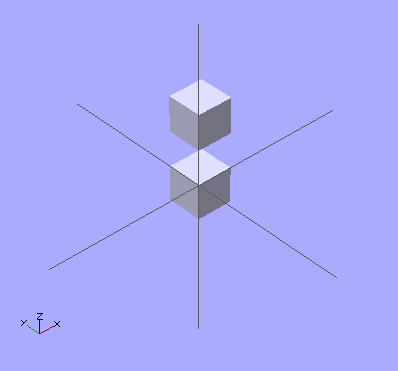Usage example 2a - iteration over a range: for ( i = [0 : 5] ){     rotate( i * 360 / 6, [1, 0, 0])     translate([0, 10, 0])     sphere(r = 1);}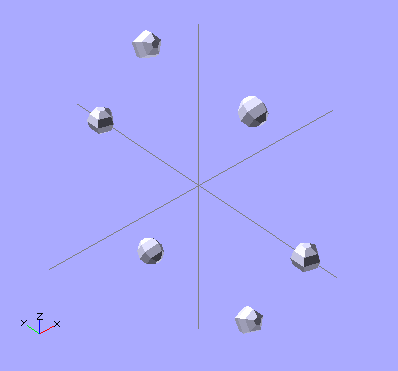Usage example 2b - iteration over a range specifying an increment: // Note: The middle parameter in the range designation // ('0.2' in this case) is the 'increment-by' value// Warning: Depending on the 'increment-by' value, the// real end value may be smaller than the given one.for ( i = [0 : 0.2 : 5] ){     rotate( i * 360 / 6, [1, 0, 0])     translate([0, 10, 0])     sphere(r = 1);}
 Usage example 3 - iteration over a vector of vectors (rotation): for(i = [ [  0,  0,   0],           [ 10, 20, 300],           [200, 40,  57],           [ 20, 88,  57] ]){     rotate(i)     cube([100, 20, 20], center = true);}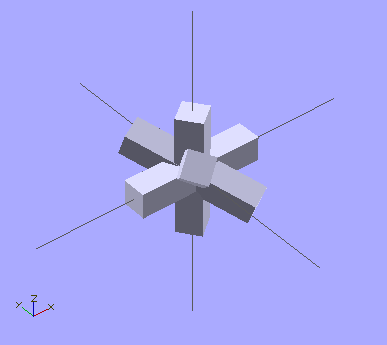Usage example 4 - iteration over a vector of vectors (translation):  for(i = [ [ 0,  0,  0],            [10, 12, 10],            [20, 24, 20],            [30, 36, 30],            [20, 48, 40],            [10, 60, 50] ]){     translate(i)     cube([50, 15, 10], center = true);}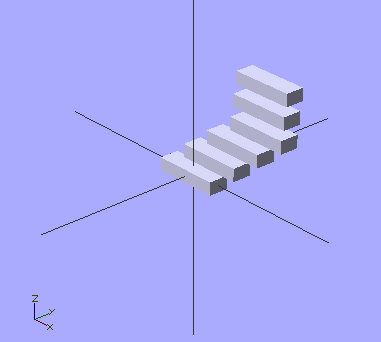Nested loop example

  for (xpos=[0:3], ypos = [2,4,6]) // do twelve iterations, using each xpos with each ypos
translate([xpos*ypos, ypos, 0]) cube([0.5, 0.5, 0.5]);

### Intersection For Loop

Iterate over the values in a vector or range and take an intersection of the contents.

Note: intersection_for() is a work around because of an issue that you cannot get the expected results using a combination of the standard for() and intersection() statements. The reason is that for() do a implicit union() of the contents.

Parameters

• <loop variable name>

• Name of the variable to use within the for loop.

 Usage example 1 - loop over a range: intersection_for(n = [1 : 6]){     rotate([0, 0, n * 60])     {         translate([5,0,0])         sphere(r=12);     }}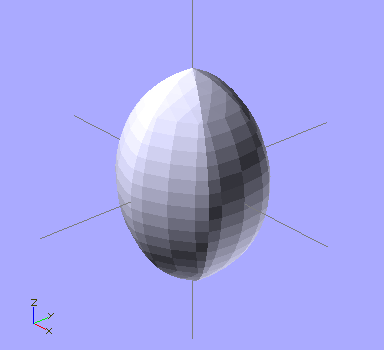Usage example 2 - rotation :  intersection_for(i = [ [  0,  0,   0],   [ 10, 20, 300],   [200, 40,  57],   [ 20, 88,  57] ]){     rotate(i)     cube([100, 20, 20], center = true);}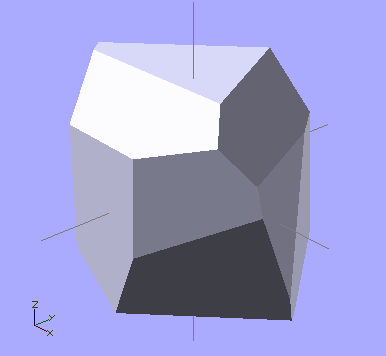### If Statement

Conditionally evaluate a sub-tree.

Parameters

• The boolean expression that should be used as condition

NOTE:

Do not confuse the assignment operator '=' with the equal operator '=='

 if (a=b) dosomething();  // WRONG - this will FAIL to be processed without any error message
if (a==b) dosomething(); // CORRECT - this will do something if a equals b

NOTE:

Assignment is not allowed within either branch of an if statement. Consider using the ternary operator 'condition ? consequent: alternative'.

// WRONG - this will FAIL to be processed with a syntax error messageif (condition){
x = consequent;} else {
x = alternative;}

// CORRECT - this will set 'x' to either 'consequent' or 'alternative'x = condition ? consequent : alternative;

Usage example:

if (x > y){
cube(size = 1, center = false);} else {
cube(size = 2, center = true);}

### Assign Statement

Set variables to a new value for a sub-tree.

Parameters

• The variables that should be (re-)assigned

Usage example:

for (i = [10:50]){
assign (angle = i*360/20, distance = i*10, r = i*2)
{
rotate(angle, [1, 0, 0])
translate([0, distance, 0])
sphere(r = r);
}}

Update: [Note: Requires version 2014.QX(see )]

Starting with this version, assign() has been deprecated as it is no longer needed; variables can be assigned anywhere.

##Mathematical OperatorsThe text in its current form is incomplete.

### Scalar Arithmetical Operators

The scalar arithmetical operators take numbers as operands and produce a new number.

 + add - subtract * multiply / divide % modulo

The "-" can also be used as prefix operator to negate a number.

### Relational Operators

All relational operator take numbers as operands and produce a Boolean value. The equal and not-equal operators can also compare Boolean values.

 < less than <= less equal == equal != not equal >= greater equal > greater than

### Logical Operators

All logical operators take Boolean values as operands and produce a Boolean value.

 && Logical AND || Logical OR ! Logical NOT

### Conditional Operator

The ?: operator can be used to conditionally evaluate one or another expression. It works like the ?: operator from the family of C-like programming languages.

 ? : Conditional operator
 Usage Example: a=1;b=2;c= a==b ? 4 : 5;If a equals b, then c is set to 4, else c is set to 5.The part "a==b" must be something that evaluates to a boolean value.

### Vector-Number Operators

The vector-number operators take a vector and a number as operands and produce a new vector.

 * multiply all vector elements by number / divide all vector elements by number

### Vector Operators

The vector operators take vectors as operands and produce a new vector.

 + add element-wise - subtract element-wise

The "-" can also be used as prefix operator to element-wise negate a vector.

### Vector Dot-Product Operator

The vector dot-product operator takes two vectors as operands and produces a scalar.

 * sum of vector element products

### Matrix Multiplication

Multiplying a matrix by a vector, vector by matrix and matrix by matrix

 * matrix/vector multiplication

##Mathematical FunctionsThe text in its current form is incomplete.

## Trigonometric Functions

The trig functions use the C Language mathematics functions, which are based in turn on Binary Floating Point mathematics, which use approximations of Real Numbers during calculation. OpenSCAD's math functions use the C++ 'double' type, inside Value.h/Value.cc,

A good resource for the specifics of the C library math functions, such as valid inputs/output ranges, can be found at the Open Group website math.h & acos

### cos

Mathematical cosine function of degrees. See Cosine

Parameters

• <degrees>

• Decimal. Angle in degrees.

 Usage Example:  for(i=[0:36])     translate([i*10,0,0])        cylinder(r=5,h=cos(i*10)*50+60);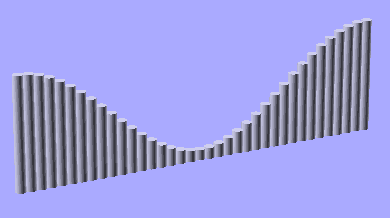### sin

Mathematical sine function. See Sine

Parameters

• <degrees>

• Decimal. Angle in degrees.

 Usage example 1:  for (i = [0:5]) {   echo(360*i/6, sin(360*i/6)*80, cos(360*i/6)*80);    translate([sin(360*i/6)*80, cos(360*i/6)*80, 0 ])     cylinder(h = 200, r=10);  }

 Usage example 2:  for(i=[0:36])     translate([i*10,0,0])        cylinder(r=5,h=sin(i*10)*50+60);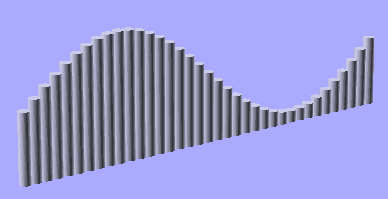### tan

Mathematical tangent function. See Tangent

Parameters

• <degrees>

• Decimal. Angle in degrees.

 Usage example:  for (i = [0:5]) {   echo(360*i/6, tan(360*i/6)*80);    translate([tan(360*i/6)*80, 0, 0 ])     cylinder(h = 200, r=10);  }

### acos

Mathematical arccosine, or inverse cosine, expressed in degrees. See: Inverse trigonometric functions

### asin

Mathematical arcsine, or inverse sine, expressed in degrees. See: Inverse trigonometric functions

### atan

Mathematical arctangent, or inverse tangent, function. Returns the principal value of the arc tangent of x, expressed in degrees. See: Inverse trigonometric functions

### atan2

Mathematical two-argument atan function, taking y as its first argument. Returns the principal value of the arc tangent of y/x, expressed in degrees. See: atan2

## Other Mathematical Functions

### abs

Mathematical absolute value function. Returns the positive value of a signed decimal number.

Usage examples:

abs(-5.0);
abs(0);
abs(8.0);

Results:

5.0
0.0
8.0

### ceil

Mathematical ceiling function. ceil(x) is the smallest integer not less than x.

See: Ceil Function

echo(ceil(4.4),ceil(-4.4));     // produces ECHO: 5, -4

### concat

[Note: Requires version 2014.QX(see )]

Return a vector containing the arguments.

Where an argument is a vector the elements of the vector are individually added to the result vector. Strings are distinct from vectors in this case.

Usage examples:

echo(concat("a","b","c","d","e","f"));          // produces ECHO: ["a", "b", "c", "d", "e", "f"]
echo(concat(["a","b","c"],["d","e","f"]));      // produces ECHO: ["a", "b", "c", "d", "e", "f"]
echo(concat(1,2,3,4,5,6));                      // produces ECHO: [1, 2, 3, 4, 5, 6]

Vector of vectors

echo(concat([ , ], [  ]));             // produces ECHO: [, , ]

Contrast with strings

echo(concat([1,2,3],[4,5,6]));                   // produces ECHO: [1, 2, 3, 4, 5, 6]
echo(concat("abc","def"));                       // produces ECHO: ["abc", "def"]
echo(str("abc","def"));                          // produces ECHO: "abcdef"

### cross

Calculates the cross product of two vectors in 3D space. The result is a vector that is perpendicular to both of the input vectors.

Using invalid input parameters (e.g. vectors with a length different from 3 or other types) will produce an undefined result.

Usage examples:

echo(cross([2, 3, 4], [5, 6, 7]));     // produces ECHO: [-3, 6, -3]
echo(cross([2, 1, -3], [0, 4, 5]));    // produces ECHO: [17, -10, 8]
echo(cross([2, 3, 4], "5"));           // produces ECHO: undef

### exp

Mathematical exp function. Returns the base-e exponential function of x, which is the number e raised to the power x. See: Exponent

echo(exp(1),exp(ln(3)*4));    // produces ECHO: 2.71828, 81

### floor

Mathematical floor function. floor(x) = is the largest integer not greater than x

See: Floor Function

echo(floor(4.4),floor(-4.4));    // produces ECHO: 4, -5

### ln

Mathematical natural logarithm. See: Natural logarithm

### len

Mathematical length function. Returns the length of an array, a vector or a string parameter.

Usage examples:

str1="abcdef"; len_str1=len(str1);
echo(str1,len_str1);

a=6; len_a=len(a);
echo(a,len_a);

array1=[1,2,3,4,5,6,7,8]; len_array1=len(array1);
echo(array1,len_array1);

array2=[[0,0],[0,1],[1,0],[1,1]]; len_array2=len(array2);
echo(array2,len_array2);

len_array2_2=len(array2);
echo(array2,len_array2_2);

Results:

ECHO: "abcdef", 6
ECHO: 6, undef
ECHO: [1, 2, 3, 4, 5, 6, 7, 8], 8
ECHO: [[0, 0], [0, 1], [1, 0], [1, 1]], 4
ECHO: [1, 0], 2

This function allows (e.g.) the parsing of an array, a vector or a string.

Usage examples:

str2="4711";
for (i=[0:len(str2)-1])
echo(str("digit ",i+1,"  :  ",str2[i]));

Results:

ECHO: "digit 1  :  4"
ECHO: "digit 2  :  7"
ECHO: "digit 3  :  1"
ECHO: "digit 4  :  1"

Note that the len() function is not defined when a simple variable is passed as the parameter.

This is useful when handling parameters to a module, similar to how shapes can be defined as a single number, or as an [x,y,z] vector; i.e. cube(5) or cube([5,5,5])

For example

module doIt(size) {
if (len(size) == undef) {
// size is a number, use it for x,y & z. (or could be undef)
do([size,size,size]);
} else {
// size is a vector, (could be a string but that would be stupid)
do(size);
}
}

doIt(5);        // equivalent to [5,5,5]
doIt([5,5,5]);  // similar to cube(5) v's cube([5,5,5])

### let

[Note: Requires version 2014.QX(see )]

Sequential assignment of variables inside an expression. The following expression is evaluated in context of the let assignments and can use the variables. This is mainly useful to make complicated expressions more readable by assigning interim results to variables.

Parameters

let (var1 = value1, var2 = f(var1), var3 = g(var1, var2)) expression

Usage Example:

echo(let(a = 135, s = sin(a), c = cos(a)) [ s, c ]); // ECHO: [0.707107, -0.707107]

### log

Mathematical logarithm. See: Logarithm

### lookup

Look up value in table, and linearly interpolate if there's no exact match. The first argument is the value to look up. The second is the lookup table -- a vector of key-value pairs.

Parameters

• key

• A lookup key

• <key,value> array

• keys and values

Notes
There is a bug where out-of-range keys will return the first value in the list. Newer versions of Openscad should use the top or bottom end of the table as appropriate instead.

 Usage example: Will create a sort of 3D chart made out of cylinders of different height.  function get_cylinder_h(p) = lookup(p, [   [ -200, 5 ],   [ -50, 20 ],   [ -20, 18 ],   [ +80, 25 ],   [ +150, 2 ]   ]);    for (i = [-100:5:+100]) {   // echo(i, get_cylinder_h(i));   translate([ i, 0, -30 ]) cylinder(r1 = 6, r2 = 2, h = get_cylinder_h(i)*3);  }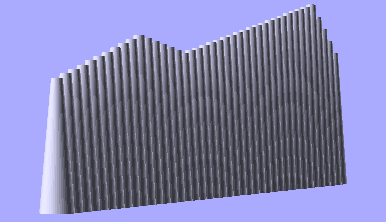### max

Returns the maximum of the parameters. If a single vector is given as parameter, returns the maximum element of that vector.

Parameters

max(n,n{,n}...)
max(vector)

• <n>

• Two or more decimals

• <vector>

• Single vector of decimals (requires OpenSCAD version 2014.06 or later).

Usage Example:

max(3.0,5.0)
max(8.0,3.0,4.0,5.0)
max([8,3,4,5])

Results:

5
8
8

### min

Returns the minimum of the parameters. If a single vector is given as parameter, returns the minimum element of that vector.

Parameters

min(n,n{,n}...)
min(vector)

• <n>

• Two or more decimals

• <vector>

• Single vector of decimals (requires OpenSCAD version 2014.06 or later).

Usage Example:

min(3.0,5.0)
min(8.0,3.0,4.0,5.0)
min([8,3,4,5])

Results:

3
3
3

Looking for mod - it's not a function, see modulo operator (%)

### norm

Returns the euclidean norm of a vector. Note this returns is the actual numeric length while len returns the number of elements in the vector or array.

Usage examples:

a=[1,2,3,4];
b="abcd";
c=[];
d="";
e=[[1,2,3,4],[1,2,3],[1,2],];
echo(norm(a)); //5.47723
echo(norm(b)); //undef
echo(norm(c)); //0
echo(norm(d)); //undef
echo(norm(e)); //5.47723
echo(norm(e)); //3.74166
echo(norm(e)); //2.23607
echo(norm(e)); //1

Results:

ECHO: 5.47723
ECHO: undef
ECHO: 0
ECHO: undef
ECHO: 5.47723
ECHO: 3.74166
ECHO: 2.23607
ECHO: 1

### pow

Mathematical power function.

Parameters

• <base>

• Decimal. Base.

• <exponent>

• Decimal. Exponent.

Usage examples:

for (i = [0:5]) {
translate([i*25,0,0]) {
cylinder(h = pow(2,i)*5, r=10);
echo (i, pow(2,i));
}
}

echo(pow(10,2)); // means 10^2 or 10*10
// result: ECHO: 100

echo(pow(10,3)); // means 10^3 or 10*10*10
// result: ECHO: 1000

echo(pow(125,1/3)); // means 125^(0.333...) which equals calculating the cube root of 125
// result: ECHO: 5

### rands

Random number generator. Generates a constant vector of pseudo random numbers, much like an array. The numbers are doubles not integers. When generating only one number, you still call it with variable

Parameters

• min_value

• Minimum value of random number range

• max_value

• Maximum value of random number range

• value_count

• Number of random numbers to return as a vector

• seed_value (optional)

• Seed value for random number generator for repeatable results.

Usage Examples:

// get a single number
single_rand = rands(0,10,1);
echo(single_rand);

// get a vector of 4 numbers
seed=42;
random_vect=rands(5,15,4,seed);
echo( "Random Vector: ",random_vect);
sphere(r=5);
for(i=[0:3]) {
rotate(360*i/4) {
translate([10+random_vect[i],0,0])
sphere(r=random_vect[i]/2);
}
}
// ECHO: "Random Vector: ", [8.7454, 12.9654, 14.5071, 6.83435]

### round

The "round" operator returns the greatest or least integer part, respectively, if the numeric input is positive or negative.

Some examples:

round(x.5) = x+1.
round(x.49) = x.
round(-(x.5)) = -(x+1).
round(-(x.49)) = -x.

round(5.4); //-> 5
round(5.5); //-> 6
round(5.6); //-> 6

### sign

Mathematical signum function. Returns a unit value that extracts the sign of a value see: Signum function

Parameters

• <x>

• Decimal. Value to find the sign of.

Usage examples:

sign(-5.0);
sign(0);
sign(8.0);

Results:

-1.0
0.0
1.0

### sqrt

Mathematical square root function.

Usage Examples:

translate([sqrt(100),0,0])sphere(100);

##String FunctionsThe text in its current form is incomplete.

### str

Convert all arguments to strings and concatenate.

Usage examples:

number=2;
echo ("This is ",number,3," and that's it.");
echo (str("This is ",number,3," and that's it."));

Results:

ECHO: "This is ", 2, 3, " and that's it."
ECHO: "This is 23 and that's it."

### chr

[Note: Requires version 2014.QX(see )]

Convert numbers to a string containing character with the corresponding code. OpenSCAD uses Unicode, so the number is interpreted as Unicode code point. Numbers outside the valid code point range will produce an empty string.

Parameters

• chr(Number)

• Convert one code point to a string of length 1 (number of bytes depending on UTF-8 encoding) if the code point is valid.

• chr(Vector)

• Convert all code points given in the argument vector to a string.

• chr(Range)

• Convert all code points produced by the range argument to a string.

Examples

echo(chr(65), chr(97));      // ECHO: "A", "a"echo(chr(65, 97));           // ECHO: "Aa"echo(chr([66, 98]));         // ECHO: "Bb"echo(chr([97 : 2 : 102]));   // ECHO: "ace"echo(chr(-3));               // ECHO: ""echo(chr(9786), chr(9788));  // ECHO: "☺", "☼"echo(len(chr(9788)));        // ECHO: 1

Note: When used with echo() the output to the console for character codes greater than 127 is platform dependent.

### Also See search()

search() for text searching.

##Transformations

Transformation affect the child nodes and as the name implies transforms them in various ways such as moving/rotating or scaling the child. Cascading transformations are used to apply a variety of transforms to a final child. Cascading is achieved by nesting statements i.e.

rotate([45,45,45])
translate([10,20,30])
cube(10);

Transformations can be applied to a group of child nodes by using '{' & '}' to enclose the subtree e.g.

 translate([0,0,-5])    or the more compact      translate([0,0,-5]) {
{                                                 cube(10);
cube(10);                                       cylinder(r=5,h=10);
cylinder(r=5,h=10);                           }
}

As OpenSCAD uses different libraries to implement capabilities this can introduce some inconsistencies to the F5 preview behaviour of transformations. Traditional transforms (translate, rotate, scale, mirror & multimatrix) are performed using OpenGL in preview, while other more advanced transforms, such as resize, perform a CGAL operation, behaving like a CSG operation affecting the underlying object, not just transforming it. In particular this can affect the display of modifier characters, specifically "#" and "%", where the highlight may not display intuitively, such as highlighting the pre-resized object, but highlighting the post-scaled object.The text in its current form is incomplete.

### scale

Scales its child elements using the specified vector. The argument name is optional.

Usage Example:
scale(v = [x, y, z]) { ... }

cube(10);
translate([15,0,0]) scale([0.5,1,2]) cube(10);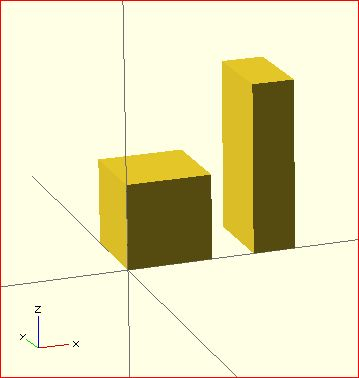### resize

resize() is available since OpenSCAD 2013.06. It modifies the size of the child object to match the given x,y, and z.

There is a bug with shrinking in the 2013.06 release, that will be fixed in the next release.

Usage Example:

// resize the sphere to extend 30 in x, 60 in y, and 10 in the z directions.
resize(newsize=[30,60,10]) sphere(r=10);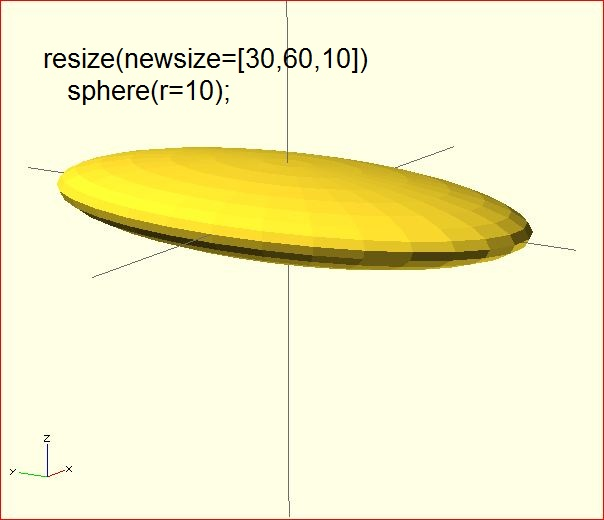If x,y, or z is 0 then that dimension is left as-is.

// resize the 1x1x1 cube to 2x2x1
resize([2,2,0]) cube();

If the 'auto' parameter is set to true, it will auto-scale any 0-dimensions to match. For example.

// resize the 1x2x0.5 cube to 7x14x3.5
resize([7,0,0], auto=true) cube([1,2,0.5]);

The 'auto' parameter can also be used if you only wish to auto-scale a single dimension, and leave the other as-is.

// resize to 10x8x1. Note that the z dimension is left alone.
resize([10,0,0], auto=[true,true,false]) cube([5,4,1]);

### rotate

Rotates its child 'a' degrees about the origin of the coordinate system or around an arbitrary axis. The argument names are optional if the arguments are given in the same order as specified.

Usage:
rotate(a = deg_a, v = [x, y, z]) { ... }
// or
rotate(deg_a, [x, y, z]) { ... }
rotate(a = [deg_x, deg_y, deg_z]) { ... }
rotate([deg_x, deg_y, deg_z]) { ... }

The 'a' argument (deg_a) can be an array, as expressed in the later usage above; when deg_a is an array, the 'v' argument is ignored. Where 'a' specifies multiple axes then the rotation is applied in the following order: x, y, z.

The optional argument 'v' is a vector and allows you to set an arbitrary axis about which the object will be rotated.

For example, to flip an object upside-down, you can rotate your object 180 degrees around the 'y' axis.

rotate(a=[0,180,0]) { ... }

This is frequently simplified to

rotate([0,180,0]) { ... }

When specifying a single axis the 'v' argument allows you to specify which axis is the basis for rotation. For example, the equivalent to the above, to rotate just around y

rotate(a=180, v=[0,1,0]) { ... }

When specifying mutiple axis, 'v'is a vector defining an arbitrary axis for rotation; this is different from the multiple axis above. For example, rotate your object 45 degrees around the axis defined by the vector [1,1,0],

rotate(a=45, v=[1,1,0]) { ... }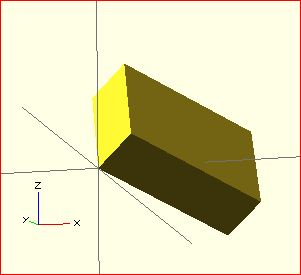Rotate with a single scalar argument rotates around the Z axis. This is useful in 2D contexts where that is the only axis for rotation. For example:

rotate(45) square(10);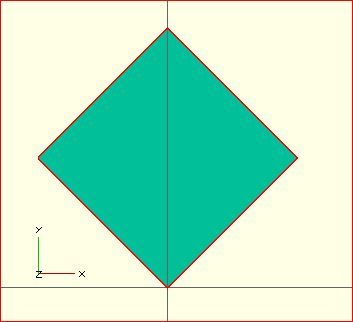##### If this is all a bit confusing, this might, or might not, help.Right-hand grip rule

For the case of:

rotate([a, b, c]) { ... };

"a" is a rotation about the X axis, from the +Z axis, toward the -Y axis. NOTE: NEGATIVE Y.
"b" is a rotation about the Y axis, from the +Z axis, toward the +X axis.
"c" is a rotation about the Z axis, from the +X axis, toward the +Y axis.

These are all cases of the Right Hand Rule. Point your right thumb along the positive axis, your fingers show the direction of rotation.

Thus if "a" is fixed to zero, and "b" and "c" are manipulated appropriately, this is the spherical coordinate system.
So, to construct a cylinder from the origin to some other point (x,y,z):

%cube(10);
x= 10; y = 10; z = 10; // point coordinates of end of cyliner
//
length = sqrt( pow(x, 2) + pow(y, 2) + pow(z, 2) );
b = acos(z/length);
c = (x==0) ? sign(y)*90 : ( (x>0) ? atan(y/x) : atan(y/x)+180 );
//
rotate([0, b, c])
cylinder(h=length, r=0.5);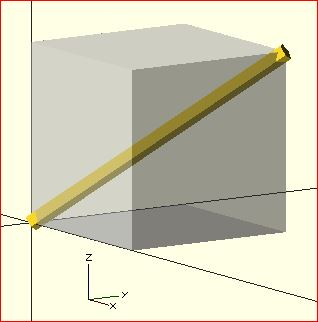### translate

Translates (moves) its child elements along the specified vector. The argument name is optional.

IExample

translate(v = [x, y, z]) { ... }

cube(2,center = true);
translate([5,0,0])
sphere(1,center = true);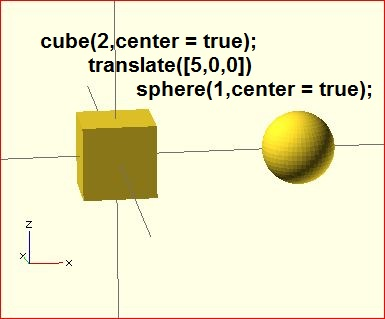### mirror

Mirrors the child element on a plane through the origin. The argument to mirror() is the normal vector of a plane intersecting the origin through which to mirror the object.

#### Function signature:

mirror( [x, y, z] ) { ... }

#### Examples

rotate([0,0,10]) cube([3,2,1]);
mirror([1,0,0]) translate([1,0,0]) rotate([0,0,10]) cube([3,2,1]);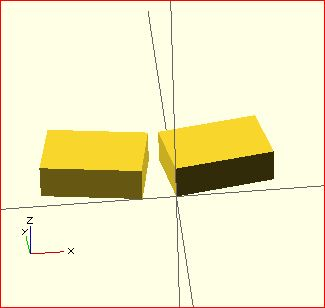### multmatrix

Multiplies the geometry of all child elements with the given 4x4 transformation matrix.

Usage: multmatrix(m = [...]) { ... }

Example (translates by [10, 20, 30]):

multmatrix(m = [ [1, 0, 0, 10],
[0, 1, 0, 20],
[0, 0, 1, 30],
[0, 0, 0,  1]
]) cylinder(r=10.0,h=10);

Example (rotates by 45 degrees in XY plane and translates by [10,20,30]):

angle=45;
multmatrix(m = [ [cos(angle), -sin(angle), 0, 10],
[sin(angle), cos(angle), 0, 20],
[0, 0, 1, 30],
[0, 0, 0,  1]
]) union() {
cylinder(r=10.0,h=10,center=false);
cube(size=[10,10,10],center=false);
}

### color

Displays the child elements using the specified RGB color + alpha value. This is only used for the F5 preview as CGAL and STL (F6) do not currently support color. The alpha value will default to 1.0 (opaque) if not specified.

#### Function signature:

color( [r, g, b, a] ) { ... }
color( [r, g, b], a=1.0 ) { ... } // since v. 2011.12 (?)
color( colorname, a=1.0 ) { ... } // since v. 2011.12 ( fails in 2014.03; use color( "colorname", #) were # is the alpha )

Note that the r, g, b, a values are limited to floating point values in the range [0,1] rather than the more traditional integers { 0 ... 255 }. However, nothing prevents you to using R, G, B values from {0 ... 255} with appropriate scaling: color([ R/255, G/255, B/255 ]) { ... }

Since version 2011.12, colors can also be defined by name (case insensitive). For example, to create a red sphere, you can write color("red") sphere(5);. Alpha is specified as an extra parameter for named colors: color("Blue",0.5) cube(5);

The available color names are taken from the World Wide Web consortium's SVG color list. A chart of the color names is as follows,
(note that both spellings of grey/gray including slategrey/slategray etc are valid):

 Purples Lavender Thistle Plum Violet Orchid Fuchsia Magenta MediumOrchid MediumPurple BlueViolet DarkViolet DarkOrchid DarkMagenta Purple Indigo DarkSlateBlue SlateBlue MediumSlateBlue Pinks Pink LightPink HotPink DeepPink MediumVioletRed PaleVioletRed
 Blues Aqua Cyan LightCyan PaleTurquoise Aquamarine Turquoise MediumTurquoise DarkTurquoise CadetBlue SteelBlue LightSteelBlue PowderBlue LightBlue SkyBlue LightSkyBlue DeepSkyBlue DodgerBlue CornflowerBlue RoyalBlue Blue MediumBlue DarkBlue Navy MidnightBlue Reds IndianRed LightCoral Salmon DarkSalmon LightSalmon Red Crimson FireBrick DarkRed
 Greens GreenYellow Chartreuse LawnGreen Lime LimeGreen PaleGreen LightGreen MediumSpringGreen SpringGreen MediumSeaGreen SeaGreen ForestGreen Green DarkGreen YellowGreen OliveDrab Olive DarkOliveGreen MediumAquamarine DarkSeaGreen LightSeaGreen DarkCyan Teal Oranges LightSalmon Coral Tomato OrangeRed DarkOrange Orange
 Yellows Gold Yellow LightYellow LemonChiffon LightGoldenrodYellow PapayaWhip Moccasin PeachPuff PaleGoldenrod Khaki DarkKhaki Browns Cornsilk BlanchedAlmond Bisque NavajoWhite Wheat BurlyWood Tan RosyBrown SandyBrown Goldenrod DarkGoldenrod Peru Chocolate SaddleBrown Sienna Brown Maroon
 Whites White Snow Honeydew MintCream Azure AliceBlue GhostWhite WhiteSmoke Seashell Beige OldLace FloralWhite Ivory AntiqueWhite Linen LavenderBlush MistyRose Grays Gainsboro LightGrey Silver DarkGray Gray DimGray LightSlateGray SlateGray DarkSlateGray Black

#### Example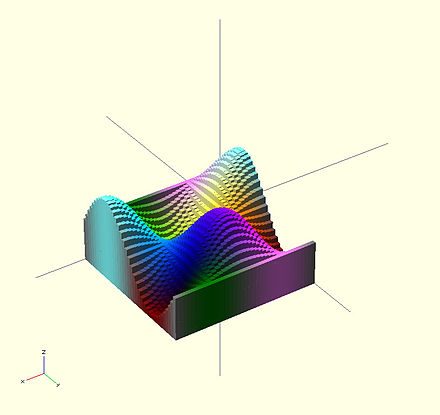A 3-D multicolor sine wave

Here's a code fragment that draws a wavy multicolor object

 for(i=[0:36]) {
for(j=[0:36]) {
color( [0.5+sin(10*i)/2, 0.5+sin(10*j)/2, 0.5+sin(10*(i+j))/2] )
translate( [i, j, 0] )
cube( size = [1, 1, 11+10*cos(10*i)*sin(10*j)] );
}
}

↗ Being that -1<=sin(x)<=1 then 0<=(1/2 + sin(x)/2)<=1 , allowing for the RGB components assigned to color to remain within the [0,1] interval.

Chart based on "Web Colors" from Wikipedia

### offset

[Note: Requires version 2014.QX(see )]

Offset allows moving polygon outlines outward or inward by a given amount.

Parameters

• r | delta

• Double. Amount to offset the polygon. When negative, the polygon is offset inwards. The parameter r specifies the radius that is used to generate rounded corners, using delta gives straight edges.

• chamfer

• Boolean. (default false) When using the delta parameter, this flag defines if edges should be chamfered or not.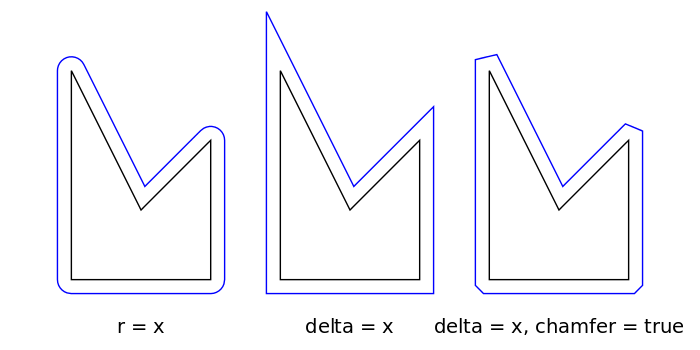Positive r/delta value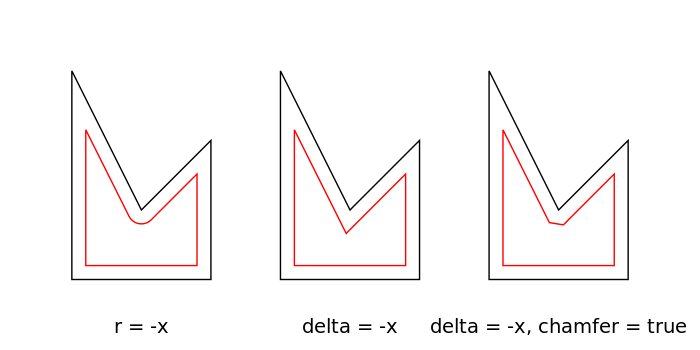Negative r/delta value

Result for different parameters. The black polygon is the input for the offset() operation.

Examples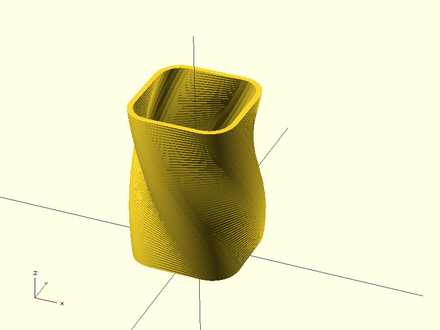Example 1: Result.

// Example 1

linear_extrude(height = 60, twist = 90, slices = 60) {
difference() {
offset(r = 10) {
square(20, center = true);
}
offset(r = 8) {
square(20, center = true);
}
}
}

### minkowski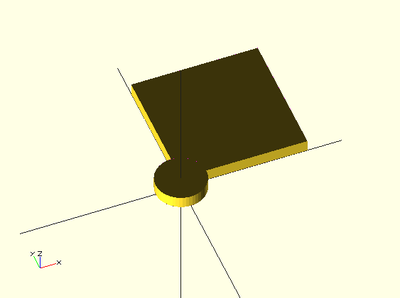A box and a cylinder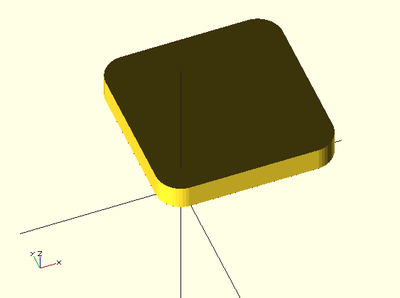Minkowski sum of the box and cylinder

Displays the minkowski sum of child nodes.

Usage example:

Say you have a flat box, and you want a rounded edge. There are many ways to do this, but minkowski is very elegant. Take your box, and a cylinder:

$fn=50; cube([10,10,1]); cylinder(r=2,h=1); Then, do a minkowski sum of them (note that the outer dimensions of the box are now 10+2+2 = 14 units by 14 units): $fn=50;
minkowski()
{
cube([10,10,1]);
cylinder(r=2,h=1);
}

### hull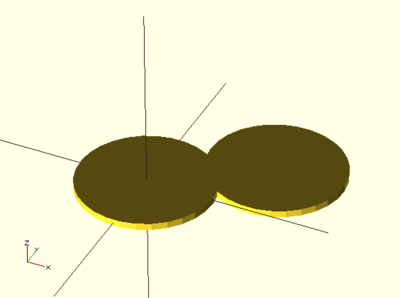Two cylinders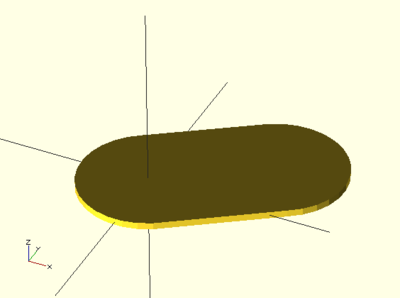Convex hull of two cylinders

Displays the convex hull of child nodes.

Usage example:

hull() {
translate([15,10,0]) circle(10);
circle(10);
}

##CSG ModelingThe text in its current form is incomplete.

### union

Creates a union of all its child nodes. This is the sum of all children.

Usage example:
union() {
cylinder (h = 4, r=1, center = true, $fn=100); rotate ([90,0,0]) cylinder (h = 4, r=0.9, center = true,$fn=100);
}

Remark: union is implicit when not used. But it is mandatory, for example, in difference to group first child nodes into one.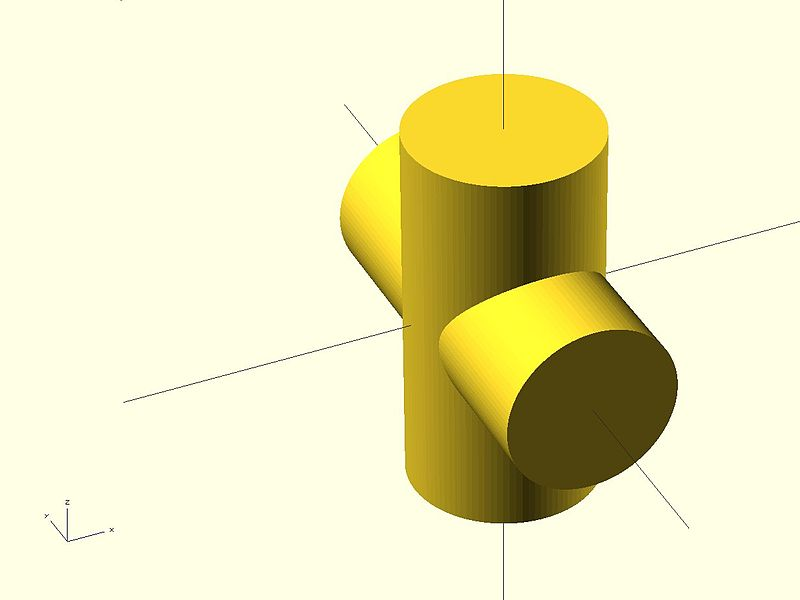### difference

Subtracts the 2nd (and all further) child nodes from the first one.

Usage example:
difference() {
cylinder (h = 4, r=1, center = true, $fn=100); rotate ([90,0,0]) cylinder (h = 4, r=0.9, center = true,$fn=100);
}

### intersection

Creates the intersection of all child nodes. This keeps the overlapping portion

Usage example:
intersection() {
cylinder (h = 4, r=1, center = true, $fn=100); rotate ([90,0,0]) cylinder (h = 4, r=0.9, center = true,$fn=100);
}

### render

Always calculate the CSG model for this tree (even in OpenCSG preview mode).

Usage example:
render(convexity = 1) { ... }

 convexity Integer. The convexity parameter specifies the maximum number of front sides (back sides) a ray intersecting the object might penetrate. This parameter is only needed for correctly displaying the object in OpenCSG preview mode and has no effect on the polyhedron rendering.

This image shows a 2D shape with a convexity of 4, as the ray indicated in red crosses the 2D shape a maximum of 4 times. The convexity of a 3D shape would be determined in a similar way. Setting it to 10 should work fine for most cases.

##Modifier CharactersThe text in its current form is incomplete.

Modifier characters are used to change the appearance or behaviours of child nodes. They are particularly useful in debugging where they can be used to highlight specific objects, or include or exclude them from rendering.

As OpenSCAD uses different libraries to implement capabilities this can introduce some inconsistencies to the F5 preview behaviour of transformations. Traditional transforms (translate, rotate, scale, mirror & multimatrix) are performed using OpenGL in preview, while other more advanced transforms, such as resize, perform a CGAL operation, behaving like a CSG operation affecting the underlying object, not just transforming it. In particular this can affect the display of modifier characters, specifically "#" and "%", where the highlight may not display intuitively, such as highlighting the pre-resized object, but highlighting the post-scaled object.

Note: The color changes triggered by character modifiers will only be shown in "Compile" mode not "Compile and Render (CGAL)" mode. (As per the color section.)

### Background Modifier

Ignore this subtree for the normal rendering process and draw it in transparent gray (all transformations are still applied to the nodes in this tree).

Because the marked subtree is completely ignored, it might have unexpected effects in case it's used for example with the first object in a difference(). In that case this object will be rendered in transparent gray, but it will not be the base for the difference()!

Usage

 % { ... }

Example

difference() {
cylinder (h = 12, r=5, center = true, $fn=100); // first object that will subtracted rotate ([90,0,0]) cylinder (h = 15, r=1, center = true,$fn=100);
// second object that will be subtracted
%rotate ([0,90,0]) cylinder (h = 15, r=3, center = true, $fn=100);} Example Output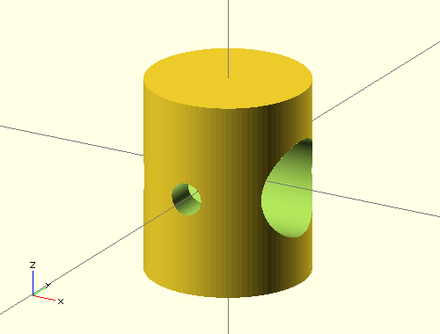Output without the modifer.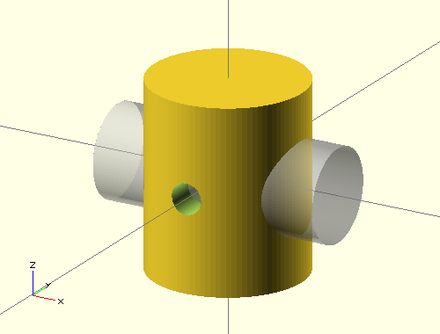Output with modifier added.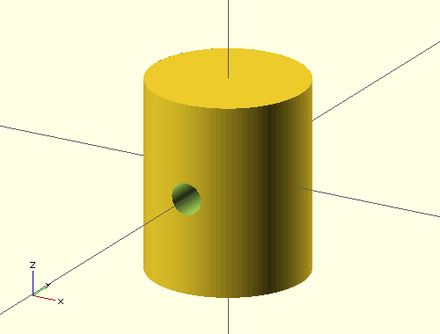Rendered Model. ### Debug Modifier Use this subtree as usual in the rendering process but also draw it unmodified in transparent pink. Usage  # { ... } Example difference() { // start objects cylinder (h = 12, r=5, center = true,$fn=100);
// first object that will subtracted
#rotate ([90,0,0]) cylinder (h = 15, r=1, center = true, $fn=100); // second object that will be subtracted #rotate ([0,90,0]) cylinder (h = 15, r=3, center = true,$fn=100);}

Example Output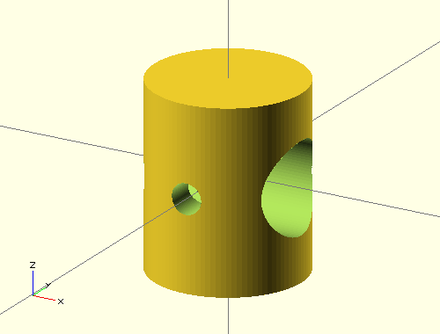Output without the modifer.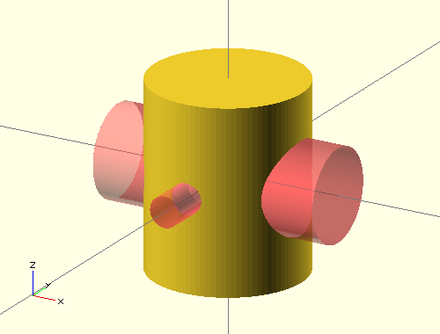### Root Modifier

Ignore the rest of the design and use this subtree as design root.

Usage

 ! { ... }

Example

difference() {
cube(10, center = true);
translate([0, 0, 5]) {
!rotate([90, 0, 0]) {
#cylinder(r = 2, h = 20, center = true, $fn = 40); } }} Example Output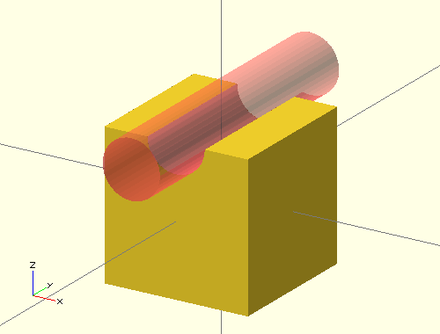Output without the modifer.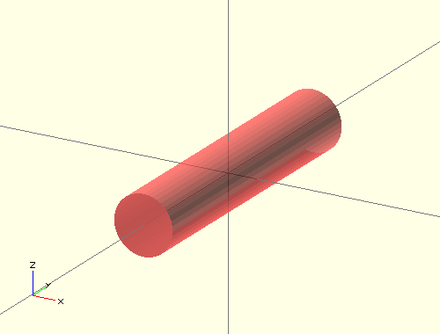Output with modifier added. As shown in the example output with the root modifier active, the rotate() is executed as it's part of the subtree marked with the root modifier, but the translate() has no effect. ### Disable Modifier Simply ignore this entire subtree. Usage  * { ... } Example difference() { cube(10, center = true); translate([0, 0, 5]) { rotate([0, 90, 0]) { cylinder(r = 2, h = 20, center = true,$fn = 40);
}
*rotate([90, 0, 0]) {
#cylinder(r = 2, h = 20, center = true, $fn = 40); } }} Example Output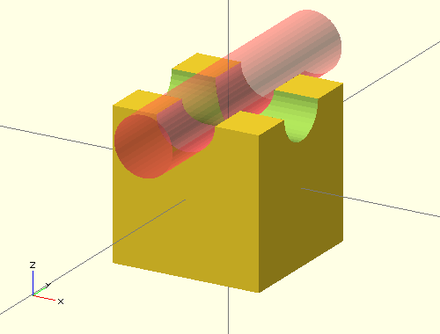Output without the modifer.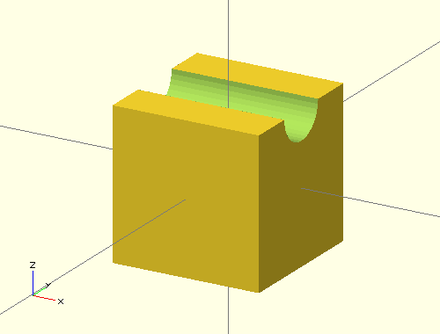Output with modifier added. The disable modifier allows to comment out one or multiple subtrees. Compared to using the usual line or multi-line comments, it's aware of the hierarchical structure which makes it easier to disable even larger trees without the need to search for the end of the subtree. ##Modules ### usage Defining your own module (roughly comparable to a macro or a function in other languages) is a powerful way to reuse procedures.  module hole(distance, rot, size) { rotate(a = rot, v = [1, 0, 0]) { translate([0, distance, 0]) { cylinder(r = size, h = 100, center = true); } } } In this example, passing in the parameters distance, rot, and size allow you to reuse this functionality multiple times, saving many lines of code and rendering your program much easier to read. You can instantiate the module by passing values (or formulas) for the parameters just like a C function call:  hole(0, 90, 10); ### children The child nodes of the module instantiation can be accessed using the children() statement within the module. The number of module children can be accessed using the$children variable.

Parameters

• empty

• select all the children

• index

• integer. select one child, at index value. Index start at 0 and should be less than or equal to $children-1. • vector • vector of integer. select children with index in vector. Index should be between 0 and$children-1.

• range

• [<start>:<end>] or [<start>:<increment>:<end>]. select children between <start> to <end>, incremented by <increment> (default 1).

Deprecated child() module

Up to release 2013.06 the now deprecated child() module was used instead. This can be translated to the new children() according to the table:

up to 2013.06 2014.03 and later
child() children(0)
child(x) children(x)
for (a = [0:$children-1]) child(a) children([0:$children-1])

Examples

Transfer all children to another module:

 // rotate to other center point:
module rz(angle, center=undef) {
translate(center)
rotate(angle)
translate(-center)
children();
}

rz(15, [10,0]) sphere(30);

Use the first child, multiple time:

 module lineup(num, space) {
for (i = [0 : num-1])
translate([ space*i, 0, 0 ]) children(0);
}

lineup(5, 65) sphere(30);

If you need to make your module iterate over all children you will need to make use of the $children variable, e.g.:  module elongate() { for (i = [0 :$children-1])
scale([10 , 1, 1 ]) children(i);
}

elongate() { sphere(30); cube([10,10,10]); cylinder(r=10,h=50); }

### arguments

One can specify default values for the arguments:

 module house(roof="flat",paint=[1,0,0]){
color(paint)
if(roof=="flat"){
translate([0,-1,0])
cube();
} else if(roof=="pitched"){
rotate([90,0,0])
linear_extrude(height=1)
polygon(points=[[0,0],[0,1],[0.5,1.5],[1,1],[1,0]],paths=[ [0,1,2,3,4] ]);
} else if(roof=="domical"){
translate([0,-1,0])
union(){
translate([0.5,0.5,1]) sphere(r=0.5,$fn=20); cube(); } } } And then use one of the following ways to supply the arguments  union(){ house(); translate([2,0,0]) house("pitched"); translate([4,0,0]) house("domical",[0,1,0]); translate([6,0,0]) house(roof="pitched",paint=[0,0,1]); translate([8,0,0]) house(paint=[0,0,0],roof="pitched"); translate([10,0,0]) house(roof="domical"); translate([12,0,0]) house(paint=[0,0.5,0.5]); } ## Importing GeometryThe text in its current form is incomplete. ### import Imports a file for use in the current OpenSCAD model Parameters • <file> • A string containing the path to the STL or DXF file. • <convexity> • An Integer. The convexity parameter specifies the maximum number of front sides (back sides) a ray intersecting the object might penetrate. This parameter is only needed for correctly displaying the object in OpenCSG preview mode and has no effect on the polyhedron rendering. Usage examples: import("example012.stl", convexity=3); import("D:\\Documents and Settings\\User\\My Documents\\Gear.stl", convexity=3); (Windows user need to backslash quote the the backslashes #### Convexity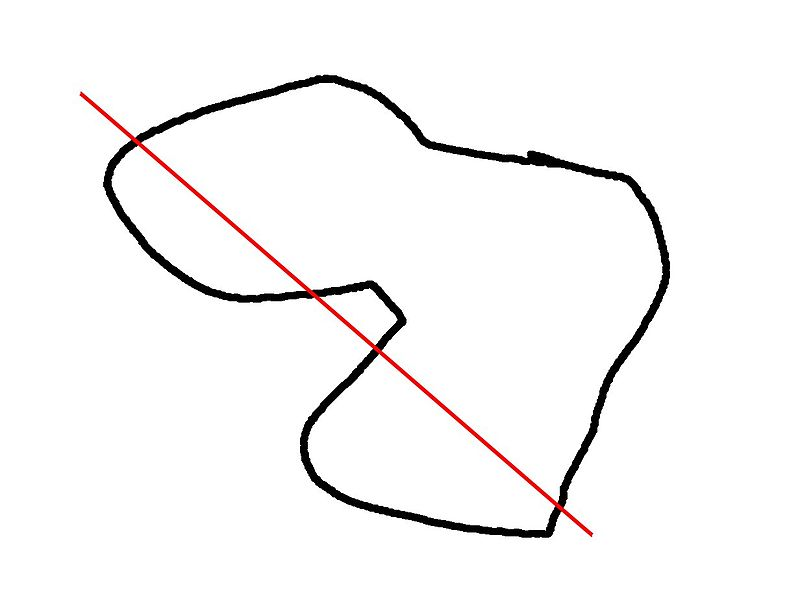This image shows a 2D shape with a convexity of 4, as the ray indicated in red crosses the 2D shape a maximum of 4 times. The convexity of a 3D shape would be determined in a similar way. Setting it to 10 should work fine for most cases. #### Notes In the latest version of OpenSCAD, import() is now used for importing both 2D (DXF for extrusion) and 3D (STL) files. If you want to render the imported STL file later, you have to make sure that the STL file is "clean". This means that the mesh has to be manifold and should not contain holes nor self-intersections. If the STL is not clean, you might get errors like:  CGAL error in CGAL_Build_PolySet: CGAL ERROR: assertion violation! Expr: check_protocoll == 0 File: /home/don/openscad_deps/mxe/usr/i686-pc-mingw32/include/CGAL/Polyhedron_incremental_builder_3.h Line: 199 or  CGAL error in CGAL_Nef_polyhedron3(): CGAL ERROR: assertion violation! Expr: pe_prev->is_border() || !internal::Plane_constructor<Plane>::get_plane(pe_prev->facet(),pe_prev->facet()->plane()).is_degenerate() File: /home/don/openscad_deps/mxe/usr/i686-pc-mingw32/include/CGAL/Nef_3/polyhedron_3_to_nef_3.h Line: 253 In order to clean the STL file, you have the following options: - use http://wiki.netfabb.com/Semi-Automatic_Repair_Options . This will repair the holes but not the self-intersections. - use netfabb basic. This free software doesnt have the option to close holes nor can it fix the self-intersections - use MeshLab, This free software can fix all the issues Using MeshLab, you can do: - Render - Show non Manif Edges - Render - Show non Manif Vertices - if found, use Filters - Selection - Select non Manifold Edges or Select non Manifold Vertices - Apply - Close. Then click button 'Delete the current set of selected vertices...' or check http://www.youtube.com/watch?v=oDx0Tgy0UHo for an instruction video. The screen should show "0 non manifold edges", "0 non manifold vertices" Next, you can click the icon 'Fill Hole', select all the holes and click Fill and then Accept. You might have to redo this action a few times. Use File - Export Mesh to save the STL. ### import_stl <DEPRECATED.. Use the command import instead..> Imports an STL file for use in the current OpenSCAD model Parameters • <file> • A string containing the path to the STL file to include. • <convexity> • Integer. The convexity parameter specifies the maximum number of front sides (back sides) a ray intersecting the object might penetrate. This parameter is only needed for correctly displaying the object in OpenCSG preview mode and has no effect on the polyhedron rendering. Usage examples: import_stl("example012.stl", convexity = 5); ## Include Statement For including code from external files in OpenSCAD, there are two commands available: • include <filename> acts as if the contents of the included file were written in the including file, and • use <filename> imports modules and functions, but does not execute any commands other than those definitions. Library files are searched for in the same folder as the design was open from, or in the library folder of the OpenSCAD installation. You can use a relative path specification to either. If they lie elsewhere you must give the complete path. Newer versions have predefined user libraries, see the OpenSCAD_User_Manual/Libraries page, which also documents a number of library files included in OpenSCAD. Windows and Linux/Mac use different separators for directories. Windows uses \, e.g. directory\file.ext, while the others use /, e.g. directory/file.ext. This could lead to cross platform issues. However OpenSCAD on Windows correctly handles the use of /, so using / in all include or use statements will work on all platforms. Using include <filename> allows default variables to be specified in the library. These defaults can be overridden in the main code. An openscad variable only has one value during the life of the program. When there are multiple assignments it takes the last value, but assigns when the variable is first created. This has an effect when assigning in a library, as any variables which you later use to change the default, must be assigned before the include statement. See the second example below. A library file for generating rings might look like this (defining a function and providing an example): ring.scad: module ring(r1, r2, h) { difference() { cylinder(r = r1, h = h); translate([ 0, 0, -1 ]) cylinder(r = r2, h = h+2); } } ring(5, 4, 10); Including the library using include <ring.scad>; rotate([90, 0, 0]) ring(10, 1, 1); would result in the example ring being shown in addition to the rotated ring, but use <ring.scad>; rotate([90, 0, 0]) ring(10, 1, 1); only shows the rotated ring. If using the use function, make sure to place the use statements at top of the file, or at least not within a module! This will work fine:  // a.scad use <ring.scad>; module a() { ring(); } but this will result in an syntax error:  //a.scad module a() { use <ring.scad>; ring(); } Default variables in an include can be overridden, for example lib.scad i=1; k=3; module x() { echo("hello world"); echo("i=",i,"j=",j,"k=",k); } hello.scad j=4; include <lib.scad>; x(); i=5; x(); k=j; x(); Produces the following ECHO: "hello world" ECHO: "i=", 5, "j=", 4, "k=", 4 ECHO: "hello world" ECHO: "i=", 5, "j=", 4, "k=", 4 ECHO: "hello world" ECHO: "i=", 5, "j=", 4, "k=", 4 However, placing j=4; after the include fails, producing ECHO: "hello world" ECHO: "i=", 5, "j=", 4, "k=", undef ECHO: "hello world" ECHO: "i=", 5, "j=", 4, "k=", undef ECHO: "hello world" ECHO: "i=", 5, "j=", 4, "k=", undef ##Other Language FeaturesThe text in its current form is incomplete. ### Special variables All variables starting with a '$' are special variables. The semantic is similar to the special variables in lisp: they have dynamic instead of lexical scoping.

What that means is that they're effectively automatically passed onward as arguments. Comparing a normal with a special variable:

     normal=2;
module doesnt_pass_it()
{   echo(normal); }
module normal_mod()
{   doesnt_pass_it(); }
normal_mod(normal=1); //Should echo 2

$special=3;$another=5;
module passes_it()
{   echo($special,$another); }
module special_mod()
{   $another=6; passes_it(); } special_mod($special=4); //Should echo 4,6

So basically it is useful when you do not want to pass many parameters all the time.

#### $fa,$fs and $fn The$fa, $fs and$fn special variables control the number of facets used to generate an arc:

$fa is the minimum angle for a fragment. Even a huge circle does not have more fragments than 360 divided by this number. The default value is 12 (i.e. 30 fragments for a full circle). The minimum allowed value is 0.01. Any attempt to set a lower value will cause a warning.$fs is the minimum size of a fragment. Because of this variable very small circles have a smaller number of fragments than specified using $fa. The default value is 2. The minimum allowed value is 0.01. Any attempt to set a lower value will cause a warning.$fn is usually 0. When this variable has a value greater than zero, the other two variables are ignored and full circle is rendered using this number of fragments. The default value is 0.

When $fa and$fs are used to determine the number of fragments for a circle, then OpenSCAD will never use fewer than 5 fragments.

This is the C code that calculates the number of fragments in a circle:

      int get_fragments_from_r(double r, double fn, double fs, double fa)
{
if (r < GRID_FINE) return 3;
if (fn > 0.0) return (int)(fn >= 3 ? fn : 3);
return (int)ceil(fmax(fmin(360.0 / fa, r*2*M_PI / fs), 5));
}

Spheres are first sliced into as many slices as the number of fragments being used to render a circle of the sphere's radius, and then every slice is rendered into as many fragments as are needed for the slice radius. You might have recognized already that the pole of a sphere is usually a pentagon. This is why.

The number of fragments for a cylinder is determined using the greater of the two radii.

The method is also used when rendering circles and arcs from DXF files.

You can generate high resolution spheres by resetting the $fX values in the instantiating module: $fs = 0.01;
sphere(2);

or simply by passing the special variable as parameter:

      sphere(2, $fs = 0.01); You can even scale the special variable instead of resetting it:  sphere(2,$fs = $fs * 0.01); ####$t

The $t variable is used for animation. If you enable the animation frame with view->animate and give a value for "FPS" and "Steps", the "Time" field shows the current value of$t. With this information in mind, you can animate your design. The design is recompiled every 1/"FPS" seconds with $t incremented by 1/"Steps" for "Steps" times, ending at either$t=1 or $t=1-1/steps. If "Dump Pictures" is checked, then images will be created in the same directory as the .scad file, using the following$t values, and saved in the following files:

• $t=0/Steps filename="frame00001.png" •$t=1/Steps filename="frame00002.png

• $t=2/Steps filename="frame00003.png" • . . . •$t=1-3/Steps filename="frame<Steps-2>.png"

• $t=1-2/Steps filename="frame<Steps-1>.png" •$t=1-1/Steps filename="frame00000.png"

Or, for other values of Steps, it follows this pattern:

• $t=0/Steps filename="frame00001.png" •$t=1/Steps filename="frame00002.png

• $t=2/Steps filename="frame00003.png" • . . . •$t=1-3/Steps filename="frame<Steps-2>.png"

• $t=1-2/Steps filename="frame<Steps-1>.png" •$t=1-1/Steps filename="frame<Steps-0>.png"

• $t=1-0/Steps filename="frame00000.png" Which pattern it chooses appears to be an unpredictable, but consistent, function of Steps. For example, when Steps=4, it follows the first pattern, and outputs a total of 4 files. When Steps=3, it follows the second pattern, and also outputs 4 files. It will always output either Steps or Steps+1 files, though it may not be predictable which. When finished, it will wrap around and recreate each of the files, looping through and recreating them forever. ####$vpr, $vpt and$vpd

These contain the current viewport rotation and translation and camera distance - at the time of doing the rendering. Moving the viewport does not update them. During an animation they are updated for each frame.

• $vpr shows rotation •$vpt shows translation (i.e. won't be affected by rotate and zoom)

• $vpd shows the camera distance [Note: Requires version 2014.QX(see )] Example  cube([10, 10,$vpr / 10]);

which makes the cube change size based on the view angle, if an animation loop is active (which does not need to use the $t variable) You can also make bits of a complex model vanish as you change the view. All three variables are writable but only assignments at the top-level of the main file will have an effect on the viewport. [Note: Requires version 2014.QX(see )] Example $vpr = [0, 0, $t * 360]; which allows a simple 360 degree rotation around the Z axis in animation mode. The menu command Edit - Paste Viewport Rotation/Translation copies the current value of the viewport, but not the current$vpr or $vpt. ### Echo Statements This function prints the contents to the compilation window (aka Console). Useful for debugging code. Also see the String function str(). Numeric values are rounded to 5 significant digits. The OpenSCAD console supports a subset of HTML markup language. See here for details. It can be handy to use 'variable=variable' as the expression to easily label the variables, see the example below. Usage examples: my_h=50; my_r=100; echo("This is a cylinder with h=", my_h, " and r=", my_r); echo(my_h=my_h,my_r=my_r); // shortcut cylinder(h=my_h, r=my_r); // echo("<b>Hello</b> <i>Qt!</i>"); Shows in the Console as ECHO: "This is a cylinder with h=", 50, " and r=", 100 ECHO: my_h = 50, my_r = 100 ECHO: "Hello Qt!" ### Render Forces the generation of a mesh even in preview mode. Useful when the boolean operations become too slow to track. Needs description. Usage examples: render(convexity = 2) difference() { cube([20, 20, 150], center = true); translate([-10, -10, 0]) cylinder(h = 80, r = 10, center = true); translate([-10, -10, +40]) sphere(r = 10); translate([-10, -10, -40]) sphere(r = 10); } ### Surface Surface reads Heightmap information from text or image files. Parameters • file • String. The path to the file containing the heightmap data. • center • Boolean. This determines the positioning of the generated object. If true, object is centered in X- and Y-axis. Otherwise, the object is placed in the positive quadrant. Defaults to false. • invert • Boolean. Inverts how the color values of imported images are translated into height values. This has no effect when importing text data files. Defaults to false. [Note: Requires version 2014.QX(see )] • convexity • Integer. The convexity parameter specifies the maximum number of front sides (back sides) a ray intersecting the object might penetrate. This parameter is only needed for correctly displaying the object in OpenCSG preview mode and has no effect on the final rendering. #### Text file format The format for text based heightmaps is a matrix of numbers that represent the height for a specific point. Rows are mapped to the Y-axis, columns to the X axis. The numbers must be separated by spaces or tabs. Empty lines and lines starting with a # character are ignored. #### Images [Note: Requires version 2014.QX(see )] Currently only PNG images are supported. Alpha channel information of the image is ignored and the height for the pixel is determined by converting the color value to Grayscale using the linear luminance for the sRGB color space (Y = 0.2126R + 0.7152G + 0.0722B). The gray scale values are scaled to be in the range 0 to 100. #### Examples Example 1: //surface.scad surface(file = "surface.dat", center = true, convexity = 5); %translate([0,0,5])cube([10,10,10], center =true); #surface.dat 10 9 8 7 6 5 5 5 5 5 9 8 7 6 6 4 3 2 1 0 8 7 6 6 4 3 2 1 0 0 7 6 6 4 3 2 1 0 0 0 6 6 4 3 2 1 1 0 0 0 6 6 3 2 1 1 1 0 0 0 6 6 2 1 1 1 1 0 0 0 6 6 1 0 0 0 0 0 0 0 3 1 0 0 0 0 0 0 0 0 3 0 0 0 0 0 0 0 0 0 Result: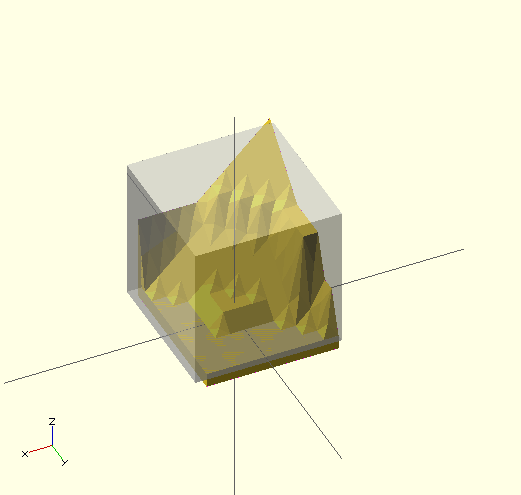Example 2  // example010.dat generated using octave: // d = (sin(1:0.2:10)' * cos(1:0.2:10)) * 10; // save("example010.dat", "d"); intersection() { surface(file = "example010.dat", center = true, convexity = 5); rotate(45, [0, 0, 1]) surface(file = "example010.dat", center = true, convexity = 5); }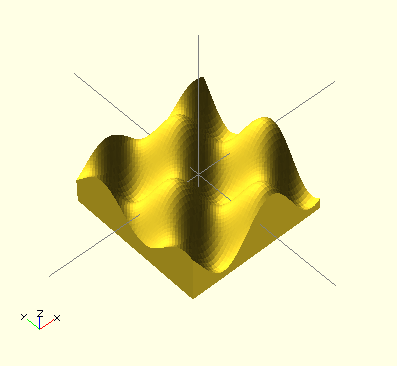Example 3: [Note: Requires version 2014.QX(see )] // Example 3a scale([1, 1, 0.1]) surface(file = "smiley.png", center = true); // Example 3b scale([1, 1, 0.1]) surface(file = "smiley.png", center = true, invert = true);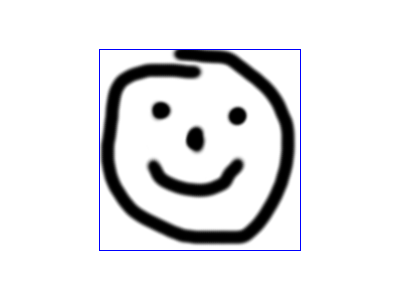Input image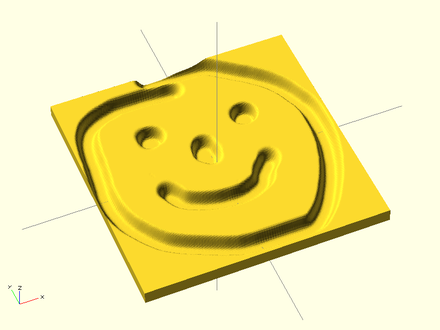Example 3a: surface(invert = false)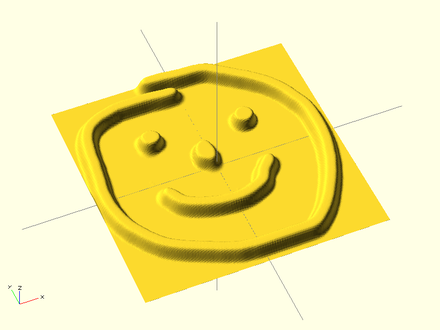Example 3b: surface(invert = true) Example 3: Using surface() with a PNG image as heightmap input. ### Search The search() function is a general-purpose function to find one or more (or all) occurrences of a value or list of values in a vector, string or more complex list-of-list construct. #### Search Usage • search( match_value , string_or_vector [, num_returns_per_match [, index_col_num ] ] ); #### Search Arguments • match_value • See Example 9 below. • Can be a single value or vector of values. • Strings are treated as vectors-of-characters to iterate over; the search function does not search for substrings. • Note: If match_value is a vector of strings, search will look for exact string matches. • string_or_vector • The string or vector to search for matches. • num_returns_per_match (default: 1) • See Example 6 below. • If num_returns_per_match = 0, search returns a list of lists of all matching index values for each element of match_value. • See Example 8 below. • By default, search only looks for one match per element of match_value to return as a list of indices • If num_returns_per_match > 1, search returns a list of lists of up to num_returns_per_match index values for each element of match_value. • index_col_num (default: 0) • When string_or_vector is a vector-of-vectors, multidimensional table or more complex list-of-lists construct, the match_value may not be found in the first (index_col_num=0) column. • See Example 5 below for a simple usage example. #### Search Usage Examples • See example023.scad included with OpenSCAD for a renderable example. ##### Index values return as list Example Code Result 1 search("a","abcdabcd");  2 search("e","abcdabcd"); [] 3 search("a","abcdabcd",0); [[0,4]] 4 search("a",[ ["a",1],["b",2],["c",3],["d",4],["a",5],["b",6],["c",7],["d",8],["e",9] ], 0); [[0,4]] (see also Example 6 below) ##### Search on different column; return Index values Example 5:  search(3,[ ["a",1],["b",2],["c",3],["d",4],["a",5],["b",6],["c",7],["d",8],["e",3] ], 0, 1); Returns:  [2,8] ##### Search on list of values Example 6: Return all matches per search vector element.  search("abc",[ ["a",1],["b",2],["c",3],["d",4],["a",5],["b",6],["c",7],["d",8],["e",9] ], 0); Returns:  [[0,4],[1,5],[2,6]] Example 7: Return first match per search vector element; special case return vector.  search("abc",[ ["a",1],["b",2],["c",3],["d",4],["a",5],["b",6],["c",7],["d",8],["e",9] ], 1); Returns:  [0,1,2] Example 8: Return first two matches per search vector element; vector of vectors.  search("abce",[ ["a",1],["b",2],["c",3],["d",4],["a",5],["b",6],["c",7],["d",8],["e",9] ], 2); Returns:  [[0,4],[1,5],[2,6],] ##### Search on list of strings Example 9:  lTable2=[ ["cat",1],["b",2],["c",3],["dog",4],["a",5],["b",6],["c",7],["d",8],["e",9],["apple",10],["a",11] ]; lSearch2=["b","zzz","a","c","apple","dog"]; l2=search(lSearch2,lTable2); echo(str("Default list string search (",lSearch2,"): ",l2)); Returns  ECHO: "Default list string search ([\"b\", \"zzz\", \"a\", \"c\", \"apple\", \"dog\"]): [1, [], 4, 2, 9, 3]" ##### Getting the right results // workout which vectors get the results v=[ ["O",2],["p",3],["e",9],["n",4],["S",5],["C",6],["A",7],["D",8] ]; // echo(v); // -> ["O",2] echo(v); // -> ["p",3] echo(v,v); // -> "p",3 echo(search("p",v)); // find "p" ->  echo(search("p",v)); // -> 1 echo(search(9,v,0,1)); // find 9 ->  echo(v[search(9,v,0,1)]); // -> ["e",9] echo(v[search(9,v,0,1)]); // -> "e" echo(v[search(9,v,0,1)]); // -> 9 echo(v[search("p",v,1,0)]); // -> 3 echo(v[search("p",v,1,0)]); // -> "p" echo(v[search("d",v,1,0)]); // "d" not found -> undef echo(v[search("D",v,1,0)]); // -> 8 ### OpenSCAD Version version() and version_num() will return OpenSCAD version number. • The version() function will return the OpenSCAD version as a vector, e.g. [2011, 09, 23] • The version_num() function will return the OpenSCAD version as a number, e.g. 20110923 ### parent_module(n) and$parent_modules

\$parent_module contains the number of modules in the instantiation stack. parent_module(i) returns the name of the module i levels above the current module in the instantiation stack. The stack is independent of where the modules are defined. It's where they're instantiated that counts. This can be used to e.g. build BOMs.

Example:

 module top() {
children();
}
module middle() {
children();
}
top() middle() echo(parent_module(0)); // prints "middle"
top() middle() echo(parent_module(1)); // prints "top"

展开阅读全文
加载中### 作者的其它热门文章

打赏
0
3 收藏
分享
打赏
0 评论
3 收藏
0
分享顶部